## Ménage à trois

In this post we will give another twist to the issue of the variables that can disturb the harmonious relationship of the couple formed by exposure and effect, so that all those dirty minds waiting else reading the title can move to the next result of Google, for sure who match what they were looking for.

We saw that there exist that are related to the effect and the exposure and how they can alter our estimates of the measures of association if these variables are not distributed evenly among the study groups. We talk about our backdoor, how to avoid it and how close it both in cohort and in case and control studies.

But sometimes the effect of exposure on the outcome studied is not always the same and can vary in intensity as the value or the level of a third variable is changed. As was the case with confounding, we observe it better stratifying the results for analysis, but in these cases is not due to the uneven distribution of the variable, but the effect of exposure is actually modified by the magnitude of this variable, which is called modifying variable or interaction effect.

Naturally, it is essential to distinguish between confounding and interaction variable. The effect of the confounding variable depends on its distribution among the study groups. In experimental studies, this distribution may vary according to the distribution occurred during randomization, so a variable can act as confounder in one trial and not in another. However, in observational studies they always exert their effect, as they are associated both with the exposure and the effect. When we find a confounding variable our goal is to control its effect and estimate an adjusted measure of association.

On the other hand, effect modifier variables represent characteristics of the relationship between exposure and effect whose intensity depends on the ménage à trois created by the interaction of this third variable. If you think about it, in the event that there’s a modification of effect we’ll not be interested in calculating an adjusted measure of association, as we could do with the Mantel-Haenszel test, because it wouldn’t be representative of the overall action of exposure on effect. Neither is good idea to do the simple arithmetic average of the measures of association we observe in each stratum. In any case what we have to do is to describe it and not try to control it, as we do with confounding variables.

Before we can say that there is an effect modifier variable we must discard that the observed differences are due to chance, confounding or bias of our study. Observing the confidence intervals of the estimated measures can help to rule out chance, as it will be more unlikely if the intervals do not overlap. We can also calculate whether differences among strata are statistically significant, using the appropriate test according to the design of the study.

And can we estimate an overall measure of the influence of exposure on the effect that takes into account the existence of an interaction variable?. Of course we can, does anyone doubt it?.

Perhaps the easiest way is to calculate a standardized measure. To do so we compare two different measures, one which assumes that each element of each stratum has the risk of the exposed and another which assumes the same but in non-exposed. Doing so we estimate a measure of the association in the global standard population we have set. Confused?. Let’s see an example. We’re going to continue boring you to exhaustion with poor smokers and their coronary artery disease. In the first table are the results of a study that I just invented over smoking and myocardial infarction.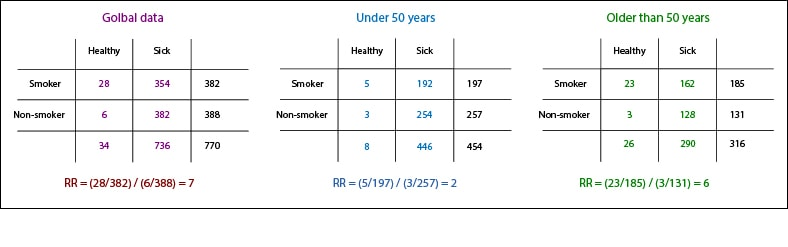We see that, overall, smokers have seven times higher risk of suffering infarction than non-smokers (relative risk, RR = 7). Let’s assume that smokers and nonsmokers have a similar age distribution, but that if we break down the data into two age groups the risks are different. The RR under 50 years is 2, compared to the older than 50, whose risk of heart attack is three times higher for smokers than for non-smokers.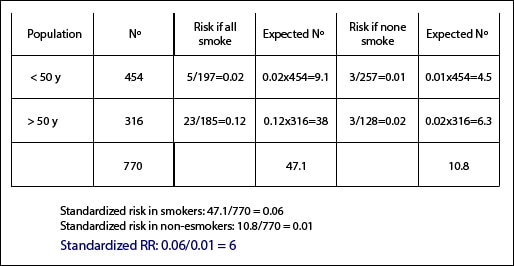We will calculate two measures of association, one assuming that everyone smokes and the other assuming none smokes. In younger than 50 years, the risk of myocardial infarction if all smoke is 5/197 = 0.02. If we have 454 people less than 50, the expected number of infarctions would be 454×0.02 = 9.1. The risk in non-smokers would be 3/257 = 0.01, so we expect to find 0.01×454 = 4.5 cases of infarction in non-smokers.

We do the same calculations with the older than 50 and we add the total number of people (770), the total number of heart attacks in smokers (47.1) and nonsmokers (10.8). The standardized risk in smokers in this population is 47.1 / 770 = 0.06. The standardized risk in nonsmokers, 10.8 / 770 = 0.01. Finally, we calculate the standardized RR: 0.06 / 0.01 = 6. This means that, globally, smoking increases six time the risk of myocardial infarction, but do not forget that this result is valid only for this standard population and it would probably not for a different population.

Just one more thing before finishing. As with the analysis of confounding variables, the analysis of effect modifiers can also be done by regression, introducing an interaction coefficient in the obtained equation to correct the effect of the modifier. Moreover, these coefficients are very useful to us because their statistical significance serves to distinguish between confusion and interaction. But that is another story…

## To what do you attribute it?

It seems like only yesterday. I began my adventures at the hospital and had my first contacts with The Patient. And, by the way, I didn’t know much about diseases but I knew without thinking about it what were the three questions with which any good clinical history began: what is bothering you?, how long has it been going on?, and to what do you attribute it?.

The fact is that the need to know the why of things is inherent to human nature and, of course, is of great importance in medicine. Everyone is mad for establishing cause and effect relations; sometimes one does it rather loosely and comes to the conclusion that the culprit of his summer’s cold is the supermarket’s guy, who has set the air conditioned at maximal power. This is the reason why studies on etiology must be conducted and assessed with scientific rigour. For this reason and because when we talk about etiology we also refer to harm, including that derived from our own actions (what educated people call iatrogenic).

This is why studies on etiology/harm have similar designs. Theis the ideal choice and we can use it, for example, to know if a treatment is the cause of the patient’s recovery. But when we study risk factors or harmful exposures, the ethical principle of nonmaleficence prevent us to randomized exposures, so we have to resort to observational studies such us cohort studies or case-control studies, although the level of evidence provided by them will be smaller than that of the experimental studies.

To critically appraise a paper on etiology / harm, we’ll resort to our well-known pillars: validity, relevance and applicability.

First, we’ll focus on the VALIDITY or scientific rigour of the work, which should answer to the question whether the factor or intervention studied was the cause of the adverse effect or disease observed.

As always, we’ll asses a series of primary validity criteria. If these are not fulfilled, we’ll left the paper and devote ourselves to something else more profitable. The first is to determine whether groups compared were similar regarding to other important factors different from the exposure studied. Randomization in clinical trials provides that the groups are homogeneous, but we cannot count on it in the case of observational studies. The homogeneity of the two cohorts is essential and the study is not valid without it. One can always argue that has stratified the differences between the two groups or that has made a multivariate analysis to control for the effect of known confounders but, what about the unknown?. The same applies to case-control studies, much more sensitive to bias and confusion.

Have exposure and effect been assessed in the same way in all groups?. In clinical trials and cohort studies we have to check that the effect has had the same likelihood of appearance and of be detected in the two groups. Moreover, in case-control studies is very important to properly asses previous exposure, so we must investigate whether there is potential bias in data collection, such us recall bias (patients often remember symptoms better than healthy). Finally, we must consider if follow-up has been long enough and complete. Losses during the study, common in observational designs, can bias the results.

If we have answered yes to all the three questions, we’ll turn to consider secondary validity criteria. Study’s results have to be evaluated to determine whether the association between exposure and effect satisfies a reasonably evidence of causality.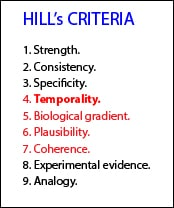One useful tool are the Hill’s criteria, which was a gentleman who suggested using a series of items to try to distinguish the causal or non-causal nature of an association. These criteria are: a) strength of association, represented by the risk ratio between exposure and effect, that we’ll consider shortly; b) consistency, which is reproducibility in populations or in different situations; c) specificity, which means that a cause produces a unique effect and no a multiple one; d) temporality: it’s essential that cause precedes the effect; e) biological gradient: the more intense the cause, the more intense the effect; f) plausibility: the relationship has to be logical according to our biological knowledge; g) coherence, the relationship should not be in conflict with other knowledge about disease or effect; h) experimental evidence, often difficult to obtain in humans for ethical reasons; and finally, i) analogy to other known situations. Although these are a quite-vintage criteria and some of them may be irrelevant (experimental evidence or analogy), they may serve as a guidance. The criterion of temporality would be a necessary one and would be well complemented with biological gradient, plausibility and coherence.

Another important aspect is to consider whether, apart from the intervention under study, both groups were treated similarly. In this type of study in which the double-blind is absent is where there is more risk of bias due to co-interventions, especially if these are treatments with a much greater effect than the exposure under study.

Regarding the RELEVANCE of the results, we must consider the magnitude and precision of the association between exposure and effect.

What was the strength of the association?. The most common measure of association is the risk ratio (RR), which can be used in trials and cohort studies. However, in case-control studies we don’t know the incidence of the effect (the effect has occurred when the study is conducted), so we used the odds ratio (OR). As we know, the interpretation of the two parameters is similar. Even the values of the two are similar when the frequency of the effect is very low. However, the greater the magnitude or frequency of the effect, the more different RR and OR are, with the peculiarity that the OR tends to overestimate the strength of the association when it is greater than 1 and underestimate it when it is less than 1. Anyway, these vagaries of OR will exceptionally modify the qualitative interpretation of the results.

It has to be kept in mind that a test is statistically significant for any value of OR or RR whose confidence interval does not include one, but observational studies have to be a little more demanding. Thus, in a cohort study we’ll like to see values greater than or equal to three for RR and equal than or greater than four in case-control studies.

Another useful parameter (in trials and cohort studies) is the difference in risks or incidence difference, which is a fancy way of calling our known absolute risk reduction (ARR), which allows us to calculate the NNT (or NNH, number needed to harm) parameter that best quantifies us the clinical significance of the association. Also, similar to the relative risk reduction (RRR), we have the attributable fraction in the exposed, which is the percentage of risk observed in the exposed that is due to exposure.

And, what is the accuracy of the results?. As we know, we’ll use our beloved confidence intervals, which serve to determine the accuracy of the parameter estimate in the population. It is always useful to have all these parameters, which must be included in the study or its calculation should be possible from the data provided by the authors.

Finally, we’ll asses the APPLICABILITY of the results to our clinical practice.

Are the results applicable to our patients?. Search to see if there are differences that advise against extrapolating results of the work to our environment. Also, consider what is the magnitude of the risk in our patients based on the results of the study and their characteristics. And finally, having all this information in mind, we must think about our working conditions, the choices we have and the patient’s preferences to decide whether to avoid or not the studied exposure. For example, if the magnitude of the risk is high and we have an effective alternative, the decision will be clear, but things are not always so simple.

As always, I advise you to use the resources available on the Internet, such as CASP’s, both the design-specific templates and the calculator to assess the relevance of the results.

Before concluding, let me clarify one thing. Although we’ve said we use RR in cohort studies and clinical trials and we use OR in case-control studies, actually we can use OR in any type of study (not so for RR, for which we must know the incidence of the effect). The problem is that ORs are somewhat less accurate, so we prefer to use RR and NNT whenever possible. However, OR is increasingly popular for another reason, its use in logistic regression models, which allow us to obtain estimates adjusted for confounding variables. But that’s another story…

## The ratio’s trap

The realm of science is full of traps. They’re everywhere. Neither the major medical journal, nor the most prestigious authors are free of them. Many people tend to take advantage of our ignorance and use interested indicators instead of using the proper ones in order to show the results in an interested way. For this reason, we have to be very alert and always look at the studies’ data to get our own interpretation.

Unfortunately, we cannot avoid the results being manipulated, but we can fight our ignorance and always do a critical appraisal when reading scientific papers.

An example of what I am talking about is the choice between risk ratio and odds ratio.

You know the difference between risk and odds. A risk is the proportion of subjects with an event in a total group of susceptible subjects. Thus, we can calculate the risk of having a heart attack among smokers (infarcted smokers divided by the total number of smokers) and among non-smokers (the same, but with non-smokers). If we go a step further, we can calculate the ratio between the two risks, called relative risk or risk ratio (RR), which indicates how much more likely is the occurrence of the event in one group compared with the other group.

Meanwhile, the odds represents a quite different concept. The odds indicates how much more likely is an event to occur than not to occur (p/(1-p)). For example, the odds of suffering a heart attack in smokers is calculated dividing the likelihood of having an attack in smokers (infarcted smokers divided by the total number of smokers, same that we did with the risk) by the probability of not suffering the attack in smokers (non-infarcted smokers divided by the total number of smokers or, equivalently, one minus the likelihood of having the attack). Like we did with the risk, we can calculate the ratio of the odds of the two groups to get the odds ratio (OR), which gives us an idea of how much more likely is the event to occur in one group than the other.

As you can see, they are similar but different concepts. In both cases, the null value is one. A value greater than one indicates that subject located in the numerator have a greater risk, whilst a value less than one indicates that they have less risk of presenting the event. Thus, a RR of 2.5 would mean that the group in the numerator has a 150% greater chance of presenting the event that we are studying. An OR of 2.5 means that it’s two and a half times more likely to present the event in the numerator’s group.

In other way, a RR of 0.4 indicates a 60% reduction of the probability of the event in the numerator group. An OR of 0.4 is more complex to interpret, but it’s more or less the same meaning.

Which of the two should we use?. It depends on the type of study. To calculate the RR we have to previously calculate the risks in the two groups, and for that we have to know the prevalence or cumulative incidence of the disease, so this measure is often used in cohort studies and clinical trials.

In the studies in which the prevalence of disease is unknown, as in case-control studies, there’s no choice but to use OR. But using OR is not restricted to this type of study. We can use it whenever we want, instead of use RR. In addition, a particular case is when it’s used a logistic regression model to adjust for the different confounding factors detected, which provide adjusted ORs.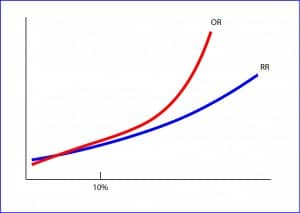In any case, RR and OR values are similar when the frequency of the effect is low, below 10%, although OR is always slightly lower than RR for values less than one and a little higher for values larger than one. Just a little?. Well, sometimes not so little. In the attached graphic it’s approximately represented the relation between RR and OR. As you can see, as the frequency of the event increases, the OR grows much faster than the RR. And here is where the trap lies, since for the same risk, the impact may seem much higher if we use an OR than if we use a RR. The OR can be misleading when the event is frequent. Let’s see an example.

Imagine that I’m very concerned with obesity among attendees to a movie theater and I want to prevent them to enter the room with a huge tank of a sugary drink whose brand I’m not going to mention. So I count how many viewers buy the drink and get a proportion of 95% of the attendees. Then, a different day, I put a sign in the bar warning about the bad health effect of drinking sugary beverages and, very gladly, I see how the proportion reduces down to an 85%.

In our case, the absolute risk measure of effect is the absolute risk difference, which is only of 10%. That’s something, but it doesn’t look like much: I only get the desired effect in one in ten. Let’s see how association measures work.

The RR is calculated as the ratio 95/85 = 1.17. This indicates that the risk of buying the drink is a 17% higher if we don’t put the sign than if we put it. It doesn’t seem too much, does it?.

The odd of buying the beverage would be 95/(1-95) without putting the sign and 85/(1-85) putting it, so the OR would be equal to (95/5)/(85/15) = 3.35. It means that it’s three times more likely to buy the beverage if we don’t put the sign.

It’s clear that RR gives a better idea that corresponds better with the absolute measure (risk difference), but now I wonder: if my brother-in-law had a factory to make signs, what indicator do you think he would use? No doubt he would use the OR.

This is why we must always look at the results to check if we can calculate some absolute indicator from the study data. Sometimes this is not as easy as in our example, as when the authors presents the OR provided by a regression model. In these cases, if we know the prevalence of the effect or disease under study, we can always calculate the equivalent RR using the following formula:And here we leave the traps for today. You have seen how data and the way of presenting them can be manipulated to say what you want without actually lying. There’re more examples of misuse of relative association measures instead of absolutes ones, such us using the relative risk difference instead of the absolute risk difference. But that’s another story…

## The one with the foreign name

Do you like to play? I’m talking about gambling and people going to casinos with the vain hope of winning a little (or no so little) money while having fun. But people who’d like to get rich in a quick and funny way forget two things. First is that everything they can see around them (and much more that they don’t see) has been paid by the thousands who previously failed in a similar attempt at the same place. Second, they forget to previously thoroughly study what are their chances of winning… and their odds.

You may wonder what an odds is. Well, to answer this question we have to warm up a few neurons.

We all understand the concept of probability. If someone ask what is the probability of getting a six when rolling a die in the casino, we’ll quickly respond that the probability is one in six or one sixth (0.16 or 16.66%). But the gambler may be interested in knowing how much more likely is to get six than not to get it. And the answer to that is not 1/6, but one fifth. Why? Because the probability of getting six is 1/6 and that of getting otherwise is 5/6. To find out how much more likely is to get six we have to divide 1/6 by 5/6, which will give us one fifth (20%). This is the odds: the probability of an event to occur respect to the probability of not to occur. For those who love formulas, odds = p / (1-p).

Let’s leave the casino for now. I have noticed that those nights that I take a look at the news on the Internet before going to bed I sleep worse. Suppose we take a survey asking people we run into on the street if they sleep well and if the use to watch the news before going to bed and we come up with the results that I show in the table.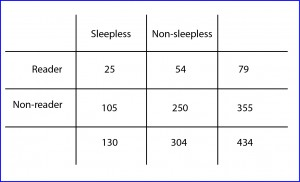We may ask what is the probability that someone who is sleepless usually read the news? Easy to answer: 25/79 or 0.31 (number of sleepless readers divided by number of readers). Moreover, what are the odds of being a sleepless reader? Also simple: the number of sleepless reader divided by the number of sleepless, or 25/54 or 0.46.

We also calculate the probability that a non-reader being sleepless as the quotient 105/355 = 0.29 (non-sleepless non-readers divided by total non-readers). The odds, meanwhile, would be 105/250 = 0.42 (sleepless non-readers divided by non-sleepless non-readers).

If we now calculate the ratio of the two probabilities we’ll get the relative risk, RR = 0.31 / 0.29 = 1.06. This means that the risk of having insomnia is more or less the same among those who read the news and those who do not. If we calculate the ratio of the two odds we’ll get a value of 1.09 (0.46/0.42). This is call the odds ratio (OR), an interesting parameter whose utility we’ll soon see.

Let’s now look again to the data in the table, but this time in reverse. What is the probability that an insomniac read the news: 25/130 = 0.19. What are the odds of insomniacs reading respect to non-reading the news: 25/105 = 0.23. What is the probability that you don’t be sleepless but be a reader: 54/304 = 0.17. And the odds: 54/250 = 0.21.

If we calculate the RR = 0.19/0.17 = 1.11, we’ll say that insomniacs have about the same risk of having read the news before going to bed than those who sleep peacefully. What about the odds? The odds is 0.23/0.58 = 1.09. What a surprise! The OR value is the same with independence of the way we manage the data, which must not be a coincidence, but must hide some meaning.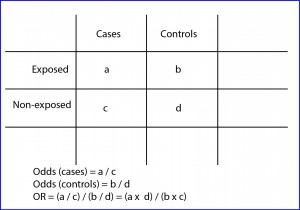And this is because the OR measures the strength of the association between the effect (insomnia) and the exposure (to read the news). Its value is always the same even if we change the orders of the ratios in the table.

As with other parameters, the correct way is to calculate confidence intervals to know the accuracy of the estimate. In addition, this association will be statistically significant if the interval does not include the value of one, which is the null value for the OR. The greater the OR the greater is the strength of the association. An OR less than one is more complex to interpret, but we can do a similar reasoning we did when RR was less than one. But here end the similarities between them. To use RR we need to know the incidence of the effects in the two populations in comparison, while the OR is calculated based on the observed frequency in the two, so they are not comparable parameters although their interpretation is similar. They tend to be equivalent only when the effect has a very low frequency of occurrence. For these reason, the OR is the measure of association used in case-control studies and meta-analysis, whereas RR are preferable for cohort studies and clinical trials.

Just a couple of considerations before finishing the issue of OR. First, although it allows us to compare the association between two qualitative variables (categorized as yes or no), it doesn’t serve to establish a cause and effect relationship between them. Second, it’s useful because it allows evaluating the effect of other variables on the association, which has a role in the realization of logistic regression studies. But that’s another story…

## Do they not trick you with cheese

If you have at home a bottle of wine that has gotten a bit chopped up, take my advice and don’t throw it away. Wait until you receive one of those scrounger visits (I didn’t mention any brother-in-law!) and offer it to drink it. But you have to combine it with a rather strong cheese. The stronger the cheese is, the better the wine will taste (you can have other thing with any excuse). Well, this trick almost as old as the human species has its parallels in the presentation of the results of scientific work.

Let’s suppose we conduct a clinical trial to test a new antibiotic (call it A) for the treatment of a serious infection that we are interesting in. We randomize the selected patients and give them the new treatment or the usual one (our control group), as chance dictates. Finally, we measure in how many of our patients there’s a treatment failure (how many has the event we want to avoid).

Thirty-six out of the 100 patients receiving drug A presented the event to avoid. Therefore, we can conclude that the risk or incidence of presenting the event in the exposed group (Ie) is 0.36 (36 out of 100). Moreover, 60 out of the 100 controls (we call them the non-exposed group) presented the event, so we quickly compute the risk or incidence in non-exposed (Io) is 0.6.

We see at first glance that risks are different in each group, but as in science we have to measure everything, we can divide risks between exposed and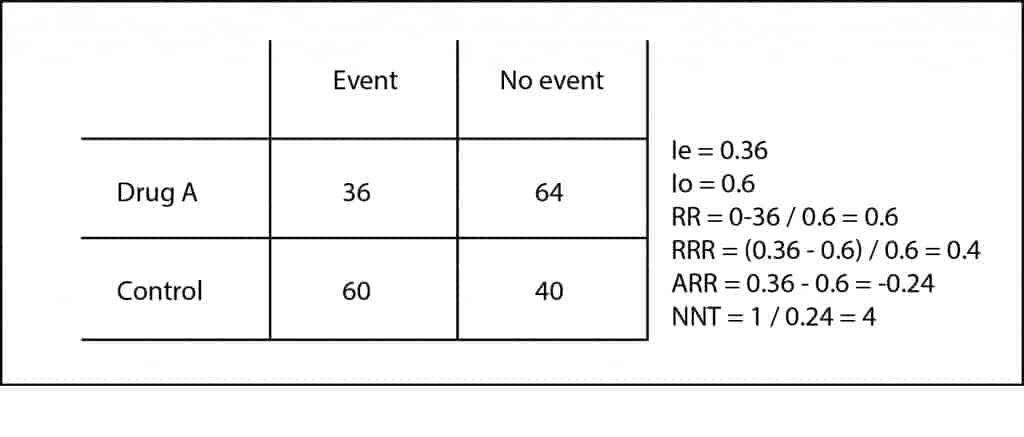non-exposed to get the so-called relative risk or risk ratio (RR = Ie/Io). A RR = 1 means that the risk is the same in both groups. If RR > 1, the event is more likely in the exposed group (and the exposure we’re studying will be a risk factor for the production of the event); and if RR is between 0 and 1, the risk will be lower in the exposed. In our case, RR = 0.36 / 0.6 = 0.6. It’s easier to interpret the RR when its value is greater than one. For example, a RR of 2 means that the probability of the event is two times higher in the exposed group. Following the same reasoning, a RR of 0.3 would tell us that the event is two-thirds less common in exposed than in controls.

But what interests us is how much decreases the risk of presenting the event with our intervention, in order to estimate how much effort is needed to prevent each event. So we can calculate the relative risk reduction (RRR) and the absolute risk reduction (ARR). The RRR is the difference in risk between the two groups with respect to the control group (RRR = [Ie-Io] / Io). In our case its value is 0.6, which mean that the tested intervention reduces the risk by 60% compared to standard therapy.

The ARR is simpler: it’s the subtraction between the exposes’ and control’s risks (ARR = Ie – Io). In our case is 0.24 (we omit the negative sign; that means that for every 100 patients treated with the new drug, it will occur 24 less events than if we had used the control therapy. But there’s more: we can know how many patients we have to treat with the new drug to prevent each event just using a rule of three (24 is to 100 as 1 is to x) or, more easily remembered, calculating the inverse of the ARR. Thus, we come up with the number needed to treat (NNT) = 1 / ARR = 4.1. In our case we would have to treat four patients to avoid an adverse event. The clinical context will tell us the clinical relevance of this figure.

As you can see, the RRR, although technically correct, tends to magnify the effect and don’t clearly quantify the effort required to obtain the result. In addition, it may be similar in different situations with totally different clinical implications. Let’s look at another example. Suppose another trial with a drug B in which we get three events in the 100 patients treated and five in the 100 controls.

If you do the calculations, the RR is 0.6 and the RRR is 0.4, as in our previous example, but if you compute the ARR you’ll come up with a very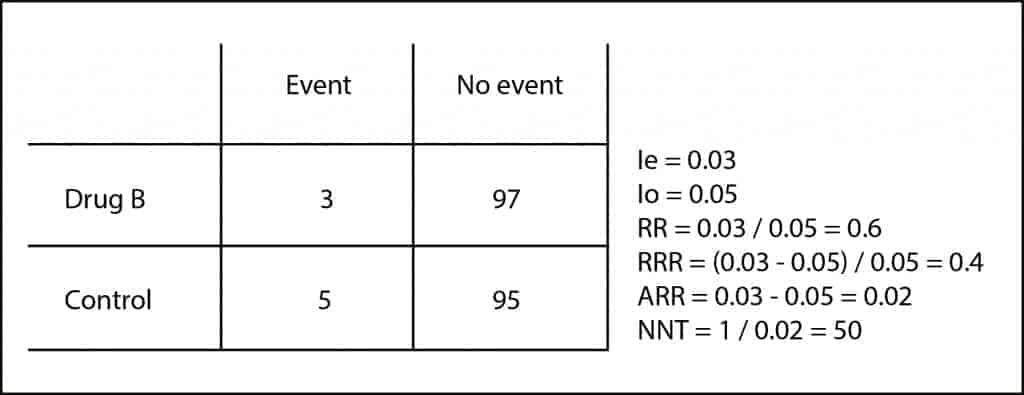different result (ARR = 0.02) and a NNT of 50. It’s clear that the effort to prevent an event is much higher (four vs. 50) despite matching the RR and RRR.

So, at this point, let me advice you. As the data needed to calculate RRR are the same than to calculate the easier ARR (and NNT), if a scientific paper offers you only the RRR and hide the ARR, distrust it and do as with the brother-in-law who offers you wine and strong cheese, asking him to offer an Iberian ham pincho. Well, I really wanted to say that you’d better ask your shelves why they don’t give you the ARR and compute it using the information from the article.

One final thought to close the topic. There’s a tendency and confusion when using or analyzing another measure of association employed in some observational studies: the odds ratio. Although they can sometimes be comparable, as when the prevalence of the effect is very small, in general, odd ratio has other meaning and interpretation. But that’s another story…

## Brown or blond, all bald

Have you ever wondered why some people go bald, especially men at a certain age?. I think it has something to do with hormones. Anyway, it’s something that the affected usually like the least, even though the popular believe that bald are smarter. It seems to me that there is nothing wrong with being bald (it’s much worse to be an asshole) but, of course, I have all my hair on my head.

Following the thread of baldness, let’s suppose we want to know if hair color has anything to do with going bald sooner or later. We set up a non-sense trial with 50 brown-hair and 50 blond-hair participants to study how many go bald and when they do it.

This example serves us to illustrate the different types of variables that we can found in a clinical trial and the different methods that we use to compare each of them.

Some variables are of quantitative continuous type. For instance, the weight of participants, their height, their income, the number of hair per square inch, etc.. Others are qualitative, such as hair color. In this case, we simplify it to a binary variable: brown or blond. Finally, there is a time-to-event type, which show the time it takes participants to present the event in study, in our case, baldness.

However, when comparing differences among these variables between the two groups of the study we have to pick out a method that will be determined by the type of variable that is being considered.

If we deal with a continuous variable such us age or weight between bald and hairy people, or between brown and blond, we’ll use the Student’s t test, provided that our data fit a normal distribution. If that is not the case, the non-parametric test that we would use is the Mann-Whitney’s.

And what if we want to compare several continuous variables at once?. Then we’ll use multiple lineal regression to make comparison among variables.

For qualitative variables the approach is different. To find out if there is a statistically significant dependence between two qualitative variables we have to build a contingency table and use the chi-squared or Fisher’s exact test, depending on our data. When in doubt, we can always use the Fisher’s test. Although it involves a more complex calculation, this is no problem for any of the statistical packages available today.

Another possibility is to calculate a measure of association, such us the relative risk or odds ratio, with its corresponding confidence interval. If the interval do not intersect the line of no-effect (the one), we can consider the association as statistically significant.

But it may happen that we want to compare several qualitative variables at once. In these cases, we’ll use a logistic regression model.

Finally, we’ll discuss the time-to-event variables, a little more complicated to compare. If we deal with a variable such as the time it takes to go bald we have to build a survival or Kaplan-Meier’s curve, which graphically shows what percentage of subjects remain at any moment without presenting the event (or the percentage that has presented it, according to the way we read it). But it could be that we want to compare the survival curves of brown and blond people to see if there are any differences in the rate at which the groups present the event of going bald. In this case we have to use the log rank test.

This method is based on the comparison between the two curves based on the differences between the observed survival and the expected survival values that we could get if there were no differences between the two groups. Remember that survival refers to the moment to present the event, not necessarily death. With this technique we get a p-value that indicates whether the difference between the two survival curves is statistically significant, but tells us nothing about the magnitude of that difference.

The case of more complex calculation is when we want to compare several variables with a time-to event-variable. For this multivariate analysis we have to use a proportional hazards regression model (Cox’s regression). This model is more complex than the previous ones but, once again, any statistical software will carry it without difficulty if we feed it with the appropriate data.

And we are going to leave the bald alone for once. We could talk more about time-to-event variables. The Kaplan-Meier’s curve gives us an idea of who is presenting the event over time, but it tells us nothing about the risk of presenting it at any given time. For that we need another indicator named hazard ratio. But that’s another story…

## The table

There’re plenty of tables. And they play a great role throughout our lives. Perhaps the first one that strikes us during our early childhood is the multiplication table. Who doesn’t long, at least the older of us, how we used to repeat like parrots that of two times one equals two, two times… until we learned it by heart?. But, as soon as we achieved mastering multiplication tables we bumped into the periodic table of the elements.  Again to memorize, this time aided by idiotic and impossible mnemonics about some Indians who Gained Bore I-don’t-know-what.

But it was through the years that we found the worst table of all: the foods composition table, with its cells full of calories. This table pursues us even in our dreams. And it’s because eating a lot have many drawbacks, most of which are found out with the aid of other table: the contingency table.

Contingency tables are used very frequently in Epidemiology to analyze the relationship among two or more variables. They consist of rows and columns. Groups by level of exposure to the study factor are usually represented in the rows, while categories that have to do with the health problem that we are investigating are usually placed in the columns. Rows and columns intersect to form cells in which the frequency of its particular combination of variables is represented.

The most common table represents two variables (our beloved 2×2 table), one dependent and one independent, but this is not always true. There may be more than two variables and, sometimes, there may be no direction of dependence between variables before doing the analysis.

Simpler 2×2 tables allow analyzing the relationship between two dichotomous variables. According to the content and the design of the study to which they belong, their cells may have slightly different meanings, just as there will be different parameters that can be calculated from the data of the table.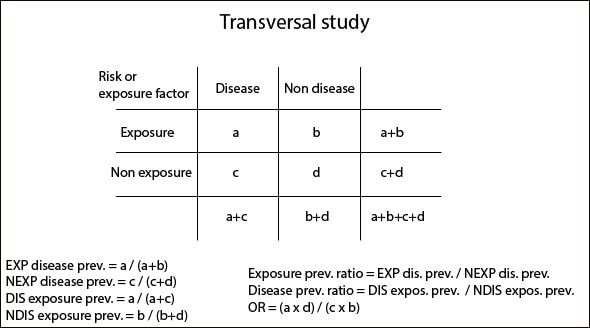The first we’re going to talk about are cross-sectional studies’ tables. This type of study represents a sort of snapshot of our sample that allows us to study the relationship between the variables. They’re, therefore, prevalence studies and, although data can be collected over a period of time, the result only represents the snapshot we have already mentioned. Dependent variable is placed in columns (disease status) and independent variable in rows (exposure status), so we can calculate a series of frequency, association and statistical significance measures.

The frequency measures are the prevalence of disease among exposed (EXP) and unexposed (NEXP) and the prevalence of exposure among diseased (DIS) and non-diseased (NDIS). These prevalences represent the number of sick, healthy, exposed and unexposed in relation to each group total, so they are rates estimated in a precise moment.

The measures of association are the rates between prevalences just aforementioned according to exposure and disease status, and the odds ratio, which tells us how much more likely the disease will occur in exposed (EXP) versus non-exposed (NEXP) people. If these parameters have a value greater than one it will indicate that the exposure factor is a risk factor for disease. On the contrary, a value equal or greater than zero and less than one will mean a protective factor. And if the value equals one, it will be neither fish nor fowl.

Finally, as in all types of tables that we’ll mention, you can calculate statistical significance measures, mainly chi-square with or without correction, Fisher’s exact test and p value, unilateral or bilateral.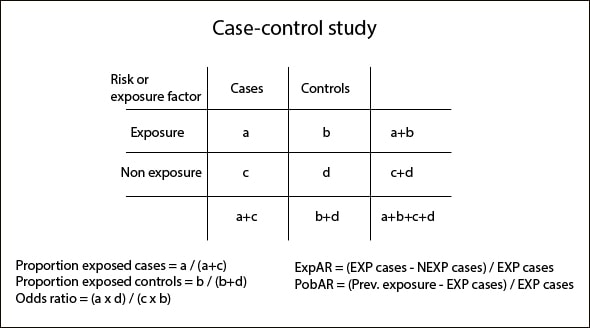Very much like those table we’ve just seen are case-control studies’ tables. This study design tries to find out if different levels of exposure can explain different levels of disease. Cases and controls are placed in columns and exposure status (EXP and NEXP) in rows.

The measures of frequency that we can calculate are the proportion of exposed cases (based on the total number of cases) and the proportion of exposed controls (based on the total number of controls). Obviously, we can also come up with the proportions of non-exposed calculating the complementary values of the aforementioned ones.

The key measure of association is the odds ratio that we already know and in which we are not going to spend much time. All of us know that, in the simplest way, we can calculate its value as the ratio of the cross products of the table and that it informs us about how much more likely is the disease to occur in exposed than in non-exposed people. The other measure of association is the exposed attributable fraction (ExpAR), which indicates the number of patients who are sick due to direct effect of exposition.

Managing this type of tables, we can also calculate a measure of impact: the population attributable fraction (PopAR), which tells us what would happen on the population if we eliminated the exposure factor. If the exposure factor is a risk factor, the impact will be positive. Conversely, if we are dealing with a protective factor, its elimination impact will be negative.

With this type of study design, the statistical significance measures will be different if we are managing paired (McNemar test) or un-paired data (chi-square, Fisher’s exact test and p value).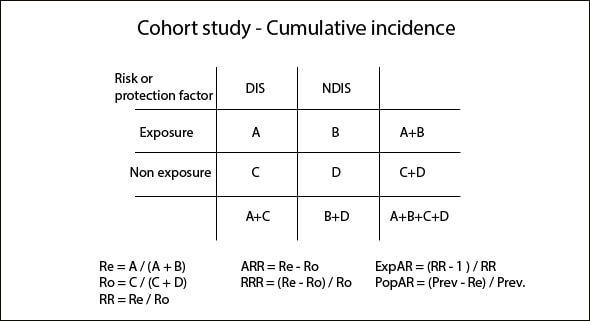The third type of contingency tables is the corresponding to cohort studies, although their structure differ slightly if you count total cases along the entire period of the study (cumulative incidence) or if you consider the time period of the study, the time of onset of disease in cases and the different time of follow-up among groups (incidence rate or incidence density).

Tables from cumulative incidence studies (CI) are similar to those we have seen so far. Disease status is represented in columns and exposure status in rows. Otherwise, incidence density (ID) tables represent in the first column the number of patients and, in the second column, the follow-up in patients-years format, so that those with longer follow-up have greater weight when calculating measures of frequency, association, etc.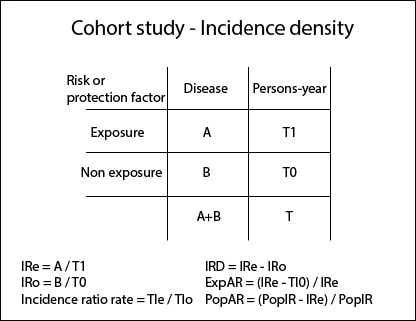The measures of frequency are the EXP risk (Re) and the NEXP risk (Ro) for CI studies and EXP and NEXP incidence rates in ID studies.

We can calculate the ratios of the above measures to come up with the association measures: relative risk (RR), absolute risk reduction (ARR) and relative risk reduction (RRR) for CI studies and incidence density reduction (IRD) for ID studies. In addition, we can also calculate ExpAR as we did in the cases-control study, as well as a measure of impact: PopAR.

We can also calculate the odds ratios if we want, but they are generally much less used in this type of study design. In any case, we know that RR and odds ratio are very similar when disease prevalence is low.

To end with this kind of table, we can calculate the statistical significance measures: chi-square, Fisher’s test and p value for CI studies and other association measures for ID studies.

As always, all these calculations can be done by hand, although I recommend you to use a calculator, such as the available one at the CASPe site. It’s easier and faster and further we will come up with all these parameters and their confidence intervals, so we can also estimate their precision.

And with this we come to the end. There’re more types of tables, with multiple levels for managing more than two variables, stratified according to different factors and so on. But that’s another story…